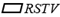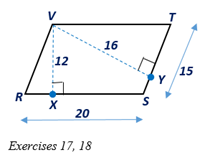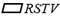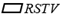Chapter 4.1, Problem 18EElementary Geometry For College St...

7th Edition
Alexander + 2 others
ISBN: 9781337614085

Solutions

Chapter
SectionElementary Geometry For College St...

7th Edition
Alexander + 2 others
ISBN: 9781337614085
Textbook Problem

In Exercises 17 and 18, considerwith V X - ⊥ R S - andV Y - ⊥ S T - .a) Which line segment isthe altitude ofwith respect to base R S - ?b) Which number is theheight ofwith respect to base R S - ?

To determine

a.

To find:

The line segment which is the altitude of RSTV respect to the base RS¯.

Explanation

Given:

Consider RSTV with VX¯RS¯ and VY¯ST¯ and the diagram as shown below.

Definition:

An altitude of a parallelogram is any line segment drawn from one side so that it is perpendicular to the nonadjacent side or to an extension of that side.

In the RSTV, given that VX¯RS¯ and VY¯ST¯

To determine

b.

To find:

The height of RSTV with to the base RS¯.

Still sussing out bartleby?

Check out a sample textbook solution.

See a sample solution

The Solution to Your Study Problems

Bartleby provides explanations to thousands of textbook problems written by our experts, many with advanced degrees!

Get Started

2. If , what asymptote does the graph of approach?

Mathematical Applications for the Management, Life, and Social Sciences

Evaluate the integral. /6/2csctcottdt

Single Variable Calculus: Early Transcendentals

True or False: If converges and converges, then converges.

Study Guide for Stewart's Multivariable Calculus, 8th